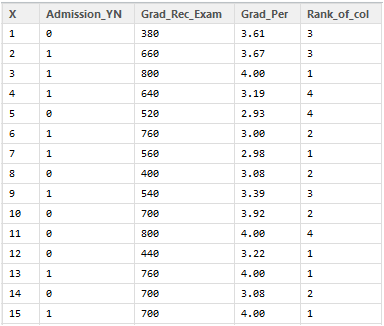# Creating, Validating and Pruning Decision Tree in R

Last updated on May 22,2019 88.1K ViewsR for Data Science is a must learn for Data Analysis & Data Science professionals. With its growth in the IT industry, there is a booming demand for skilled Data Scientists who have an understanding of the major concepts in R. One such concept, is the Decision Tree.

In this blog we will discuss :

1. How to create a decision tree for the admission data.

2. Use rattle to plot the tree.

3. Validation of decision tree using the ‘Complexity Parameter’ and cross validated error.

4. Prune the tree on the basis of these parameters to create an optimal decision tree.

To understand what are decision trees and what is the statistical mechanism behind them, you can read this post : How To Create A Perfect Decision Tree

## Creating, Validating and Pruning Decision Tree in R

To create a decision tree in R, we need to make use of the functions rpart(), or tree(), party(), etc.

rpart() package is used to create the tree. It allows us to grow the whole tree using all the attributes present in the data.

```> library("rpart")
> setwd("D://Data")
> str(data)
'data.frame': 400 obs. of 5 variables:
\$ X : int 1 2 3 4 5 6 7 8 9 10 ...
\$ Admission_YN : int 0 1 1 1 0 1 1 0 1 0 ...
\$ Grad_Rec_Exam: int 380 660 800 640 520 760 560 400 540 700 ...
\$ Grad_Per : num 3.61 3.67 4 3.19 2.93 3 2.98 3.08 3.39 3.92 ...
\$ Rank_of_col : int 3 3 1 4 4 2 1 2 3 2 ...
> View(data)``````> adm_data<-as.data.frame(data)
+ method="class")```

rpart syntax takes ‘dependent attribute’ and the rest of the attributes are independent in the analysis.

Admission_YN : Dependent Attribute. As admission depends on the factors score, rank of college, etc.

rpart() returns a Decison tree created for the data.

If you plot this tree, you can see that it is not visible, due to the limitations of the plot window in the R console.

```> plot(tree)
> text(tree, pretty=0)```## Use rattle to plot the tree:

To enhance it, let us take some help from rattle :

```> library(rattle)
> rattle()```Rattle() is one unique feature of R which is specifically built for data mining in R. It provides its own GUI apart from the R Console which makes it easier to analyze data. It has built-in graphics, which provides us better visualizations as well. Here we will use just the plotting capabilities of Rattle to achieve a decent decision tree plot.

```> library(rpart.plot)
> library(RColorBrewer)```

rpart.plot() and RcolorBrewer()  functions help us to create a beautiful plot. ‘rpart.plot()’ plots rpart models. It extends plot.rpart and text.rpart in the rpart package. RcolorBrewer() provides us with beautiful color palettes and graphics for the plots.

`> fancyRpartPlot(tree)`This was a simple and efficient way to create a Decision Tree in R. But are you sure that this is the optimal ‘Decision Tree’ for this data? If not, the following validation checks will help you.

Meanwhile, if you wish to learn R programming, check out our specially curated course by clicking on the below button.

## Validation of decision tree using the ‘Complexity Parameter’ and cross validated error :

To validate the model we use the printcp and plotcp functions. ‘CP’ stands for Complexity Parameter of the tree.

Syntax : printcp ( x ) where x is the rpart object.

This function provides the optimal prunings based on the cp value.

We prune the tree to avoid any overfitting of the data. The convention is to have a small tree and the one with least cross validated error given by printcp() function i.e. ‘xerror’.

Cross Validated Error :To find out how the tree performs, is calculated by the printcp() function, based on which we can go ahead and prune the tree.

```> printcp(tree)
Classification tree:
Variables actually used in tree construction:
Root node error: 127/400 = 0.3175
n= 400
CP nsplit rel error xerror xstd
1 0.062992 0 1.00000 1.00000 0.073308
2 0.023622 2 0.87402 0.92913 0.071818
3 0.015748 4 0.82677 0.99213 0.073152
4 0.010000 8 0.76378 1.02362 0.073760```

From the above mentioned list of cp values, we can select the one having the least cross-validated error and use it to prune the tree.

The value of cp should be least, so that the cross-validated error rate is minimum.

To select this, you can make use of this :

fit\$cptable[which.min(fit\$cptable[,”xerror”]),”CP”]

This function returns the optimal cp value associated with the minimum error.

Let us see what plotcp() function fetches.

`> plotcp(tree)`Plotcp() provides a graphical representation to the cross validated error summary. The cp values are plotted against the geometric mean to depict the deviation until the minimum value is reached.

## Prune the tree to create an optimal decision tree :

```> ptree<- prune(tree,
+ cp= tree\$cptable[which.min(tree\$cptable[,"xerror"]),"CP"])
> fancyRpartPlot(ptree, uniform=TRUE,
+ main="Pruned Classification Tree")```Thus we create a pruned decision tree.

If you wish to get a head-start on R programming, check out the Data Analytics with R course from Edureka.

Got a question for us? Please mention them in the comments section and we will get back to you.

Related Posts:

Implementing K-means clustering on the Crime dataset

Get Started with Data Science

Get Started with Business Analytics with RREGISTER FOR FREE WEBINARThank you for registering Join Edureka Meetup community for 100+ Free Webinars each month